# Numerical Modeling on Radiative Dissipative MHD Flow of a Chemically Casson Fluid over an Exponentially Inclined Stretching Surface

Numerical Modeling on Radiative Dissipative MHD Flow of a Chemically Casson Fluid over an Exponentially Inclined Stretching Surface
Department of Mathematics, GITAM (Deemed to be University), Visakhapatnam, Andhra Pradesh 530045, India

Department of Basic Sciences and Humanities, Vignan’s Institute of Engineering for Women, Andhra Pradesh 530049, India

Corresponding Author Email:
shaikmohammed.ibrahim@gitam.edu
Page:
491-501
|
DOI:
https://doi.org/10.18280/mmep.060403
2 October 2019
|
Accepted:
8 November 2019
|
Published:
24 December 2019
| Citation

OPEN ACCESS

Abstract:

This article analyzes the magnetohydrodynamic Casson nanofluid flow over an exponentially inclined stretching permeable surface considering thermal radiation, suction/injection, heat source and chemical reaction in the flow region. Mathematical formulation is developed by assuming boundary layer approach. The leading differential equations are modelled by considering similarity transformations and solved using homotopy analysis method (HAM). Parametric behaviour of various physical constraints on velocity, temperature and concentration profiles is discussed through tables and graphs. Expressions of friction factor, rate of heat and mass transfer are evaluated graphically and also in tabular form for different values of parameters. The obtained results are in fabulous agreement with the existing results. Dual solutions are presented by considering suction and injection.

Keywords:

Casson nanofluid, inclined stretching sheet, thermal radiation, viscous dissipation, HAM

1. Introduction

Heat transfer has much utilization in several branches of engineering and science, e.g. reactor cooling, nuclear waste disposal, energy production, heat conduction in tissues, etc. Extensive studies on heat transmission in non-Newtonian fluids have been done by many investigators in previous centuries. Gupta and Gupta  first analysed the characteristics of heat transfer on a stretching sheet. Corell  studied the behavior of viscous fluid flow over a nonlinear stretching sheet. Shahzad et al.  obtained the exact solution of heat transfer flow along axisymmetric nonlinear radial stretching surface. Some relevant investigations on this subject can be found in the references [4-7].

Nanoparticles can be described as particles having size between 1-100nm. Nanoparticles are a field of dominant logical attention due to its broad variety of uses in utilization of energy, toluene, microelectronic, chemical production, floor heating, minerals etc. The terminology nanofluid was induced by Choi and Eastman . Makinde and Aziz  discussed the behavior of nanofluid flow over a linear stretching sheet. Manusr and Ishak  studied the nature of a nanofluid flow past a stretching/shrinking sheet by considering convective boundary condition. Mabood et al.  derived the concept on MHD boundary layer flow of nanofluids over a nonlinear stretching sheet. Radiation effects on viscous nanofluid flow over a nonlinear stretching sheet were investigated by Hady et al. . Nadeem et al.  and Mustafa et al.  were proposed a numerical solution of non-Newtonian nanofluid over a stretching sheet. Flow and heat transfer characteristics of nanofluid over a non-linear stretching sheet using similarity solutions was carried out by Hamad and Ferdows . Chemical reaction and radiation effects on MHD mixed convection flow of a Casson nanofluid over a non-linear permeable stretching sheet was examined by Jayarami Reddy et al. . The influence of heat source and radiation on MHD stagnation point flow of Carreau nanofluid with suction and injection was analyzed by Jayarami Reddy et al. . Suneetha et al.  reported the effects of Ohmic heating and thermal radiation on MHD mixed convective flow with various parameters.

Non-Newtonian fluids have made a notable attention due to its engineering and industrial applications. Examples of these fluids are paints, suspensions, emulsions, lubricants, and many biological fluids. The common features of all these daily used products are that they do not monitor Newton’s law of viscosity. Therefore these fluids are labelled as non-Newtonian fluids. Thus, in order to study characteristics of these complex fluids, many fluid models have been suggested. These models are primarily categorized as time dependent fluids, viscoelastic fluids, time independent fluids. Out of these models, Casson fluid is one of time dependent fluid. Casson fluid model was proposed by Casson in 1959. Some samples of Casson fluid consist of honey, jelly, concentrated fruit juices and tomato sauce. Mustafa and Khan  discussed the magnetic field effect on Casson nanofluid over a nonlinearly stretching sheet. Ibrahim and Makinde  discussed the stagnation point flow of Casson nanofluid subject to slip and convective boundary conditions. Ibrahim  gave a numerical solution for the study of chemical reaction and heat source on MHD Casson nanofluid over a non-linear stretching sheet. The influence of chemical reaction and viscous dissipation on MHD mixed convection flow of Casson nanofluid over a nonlinear permeable stretching sheet was analyzed by Ibrahim et al. . Mondal et al.  analyzed the convergence stability of MHD Casson nanofluid flow with Soret and Dufour effects. Using HAM Kumar et al.  studied the 3D radiative MHD Casson nanofluid over an exponentially porous stretching sheet under convective boundary conditions.

In this paper, we study the characteristics of magnetohydrodynamic Casson nanofluid flow over an exponentially inclined stretching permeable surface using HAM. For more details on HAM, one may refer the literature done by the authors  Kumar et al. , Hayat et al. , Liao  and Nadeem et al. .

2. Mathematical Formulation

We consider two dimensional flow of incompressible viscous electrically conducting magnetohydrodynamic Casson nanofluid over an exponentially inclined stretching surface.

• $\alpha$ is an acute angle of inclination.
• The stretching velocity $U={{U}_{0}}{{e}^{x/L}}$, temperature distribution ${{T}_{w}}={{T}_{\infty }}+{{T}_{0}}{{e}^{x/L}}$ and concentration distribution ${{C}_{w}}={{C}_{\infty }}+{{C}_{0}}{{e}^{x/L}}$.
• A variable magnetic field $B={{B}_{0}}{{e}^{x/2L}}$ , ${{B}_{0}}$ is a constant is applied along the normal direction to the motion of the sheet.
• We are considering radiation, viscous dissipation and heat soure in the flow region.
• The rheological equation of state for an isotropic and incompressible flow of Casson fluid is

${{\tau }_{i\,j}}=\left[ \begin{matrix}2\left( {{\mu }_{B}}+\frac{{{p}_{y}}}{\sqrt{2\pi }} \right)\,{{e}_{i\,j}},\,\,\,\,\,\,\,\,\,\,\pi >{{\pi }_{c}}\\2\left( {{\mu }_{B}}+\frac{{{p}_{y}}}{\sqrt{2{{\pi }_{c}}}} \right)\,{{e}_{i\,j}},\,\,\,\,\,\,\,\,\,\,{{\pi }_{c}}>\pi\\\end{matrix} \right.$

where, ${{\mu }_{B}}$ is plastic dynamic viscosity of the non-Newtonian fluid, ${{p}_{y}}$ is the yield stress of the fluid, $\pi$ is the product of the component of deformation rate with itself, $\pi ={{e}_{i\,j}}{{e}_{i\,j}}$, ${{e}_{i\,j}}$ is the ${{\left( i,\,j \right)}^{th}}$ component of the deformation rate and ${{\pi }_{c}}$is a critical value of this product, based on the non-Newtonian model.

Under these assumptions the governing boundary layer equations are given by

$\frac{\partial u}{\partial x}+\frac{\partial v}{\partial y}=0,$                               (1)

\begin{align} & u\frac{\partial u}{\partial x}+v\frac{\partial u}{\partial y}=-\frac{1}{\rho }\frac{\partial p}{\partial x}+\nu \left( 1+\frac{1}{\beta } \right)\left( \frac{{{\partial }^{2}}u}{\partial {{x}^{2}}}+\frac{{{\partial }^{2}}u}{\partial {{y}^{2}}} \right) \\ & \pm g\,{{\beta }_{T}}\left( T-{{T}_{\infty }} \right)\,\cos \,\alpha +g\,{{\beta }_{c}}\left( C-{{C}_{\infty }} \right)\,\cos \,\alpha \\ & -\frac{\sigma \,B_{0}^{2}}{\rho }u-\frac{\nu }{{{K}^{*}}}u \\ \end{align}              (2)

$u\frac{\partial v}{\partial x}+v\frac{\partial v}{\partial y}=-\frac{1}{\rho }\frac{\partial p}{\partial y}+\nu \left( 1+\frac{1}{\beta } \right)\left( \frac{{{\partial }^{2}}v}{\partial {{x}^{2}}}+\frac{{{\partial }^{2}}v}{\partial {{y}^{2}}} \right)$,                          (3)

\begin{align} & u\frac{\partial T}{\partial x}+v\,\frac{\partial T}{\partial y}=\frac{k}{\rho {{c}_{p}}}\frac{{{\partial }^{2}}T}{\partial {{y}^{2}}}+\tau \left[ {{D}_{B}}\frac{\partial C}{\partial y}\,\frac{\partial T}{\partial y}+\frac{{{D}_{T}}}{{{T}_{\infty }}}{{\left( \frac{\partial T}{\partial y} \right)}^{2}} \right] \\ & +\frac{\nu }{{{c}_{p}}}\left( 1+\frac{1}{\beta } \right){{\left( \frac{\partial u}{\partial y} \right)}^{2}}-\frac{1}{\left( \rho {{c}_{p}} \right)}\frac{\partial {{q}_{r}}}{\partial y}+\frac{1}{\left( \rho {{c}_{p}} \right)}{{Q}_{0}}\left( T-{{T}_{\infty }} \right) \\ \end{align},                    (4)

$u\frac{\partial C}{\partial x}+v\frac{\partial C}{\partial y}={{D}_{B}}\frac{{{\partial }^{2}}C}{\partial {{y}^{2}}}+\frac{{{D}_{T}}}{{{T}_{\infty }}}\frac{{{\partial }^{2}}T}{\partial {{y}^{2}}}-\Gamma \left( C-{{C}_{\infty }} \right).$                               (5)

Subject to the boundary conditions

\begin{matrix} \begin{align} & u=U+N\,\mu \,\frac{\partial u}{\partial y},\,\,v=-V\left( x \right),\,\,T={{T}_{w}}+M\,\frac{\partial T}{\partial y},\, \\ & \,C={{C}_{w}}+P\,\frac{\partial C}{\partial y}\,\,at\,y=0, \\ \end{align} \\ u\to 0,\,\,\,\,T\to {{T}_{\infty }},\,\,\,\,\,\,\,C\to {{C}_{\infty }}\,\,\,\,\,\,\,\,\,\,\,as\,\,y\to \infty . \\\end{matrix}                   (6)

Here $N={{N}_{1}}\,{{e}^{-x/L}}$, $M={{M}_{1}}{{e}^{-x/L}}$, $P={{P}_{1}}{{e}^{-x/L}}$. The no-slip can be attained by assuming$N=M=P=0$ . It is assumed that  ${{K}^{*}}={{k}_{1}}{{e}^{-x/L}}$, $\Gamma ={{k}_{0}}{{e}^{x/L}}$, \tau =\frac{{{\left( \rho c \right)}_{p}}}{{{\left( \rho c \right)}_{f}}}{{q}_{r}}=-\frac{4{{\sigma }^{*}}}{3{{k}^{*}}}\frac{\partial {{T}^{4}}}{\partial y}=\frac{16\,{{\sigma }^{*}}T_{\infty }^{3}}{3\left( \rho {{c}_{p}} \right){{k}^{*}}}\frac{{{\partial }^{2}}T}{\partial {{y}^{2}}}(By Rosseland approximation), Now, we introduce the following similarity transformations to convert the partial differential equations into ordinary differential equations: \left. \begin{matrix} \begin{align} & \zeta ={{\left( \frac{{{U}_{0}}}{2\,\nu \,L} \right)}^{1/2}}{{e}^{x/2L}}y,\,\,u={{U}_{0}}\,{{e}^{x/L}}f\,'\left( \zeta \right),\, \\ & v=-\sqrt{\frac{\nu \,{{U}_{0}}}{2\,L}}{{e}^{x/2L}}\left( f\left( \zeta \right)+\zeta \,f\,'\left( \zeta \right) \right), \\ \end{align} \\ T={{T}_{\infty }}+{{T}_{0}}{{e}^{x/2L}}\theta \left( \zeta \right),C={{C}_{\infty }}+{{C}_{0}}{{e}^{x/2L}}\phi \left( \zeta \right)\, \\\end{matrix} \right\} (7) The pressure outside the boundary layer in quiescent part of flow is constant and the flow occurs only due to the stretching of the sheet and hence the pressure gradient can be neglected. Considering the usual boundary layer approximations,u>>v, \frac{\partial u}{\partial y}>>\frac{\partial u}{\partial x}, \frac{\partial v}{\partial x}, \frac{\partial v}{\partial y}, the momentum equation in y-direction reduces to\frac{\partial p}{\partial y}=0. Now substituting Eq. (7) into the Eqns. (2) to (6), we get \begin{align} & \left( 1+\frac{1}{\beta } \right)\,f\,'\,'\,'\,+f\,f\,'\,'-2\,f\,'{{\,}^{2}}\pm \lambda \,\theta \,\cos \,\alpha +\delta \,\phi \,\cos \,\alpha \\ & -\left( H+K \right)\,f\,'=0 \\ \end{align}, (8) \left( 1+\frac{4}{3}R \right)\theta '\,'+\Pr \,\left( \begin{align} & f\,\theta '-f\,'\theta +Nb\,\theta '\phi '+Nt\theta {{'}^{2}} \\ & +\left( 1+\frac{1}{\beta } \right)\,Ec\,f\,'\,{{'}^{2}}+Q\,\theta \\ \end{align} \right)\,=0, (9) \phi \,'\,'+Sc\,\left( f\,\phi '-f\,'\phi \right)+\frac{Nt}{Nb}\,\theta '\,'-Sc\,\gamma \,\phi =0. (10) The boundary conditions are \begin{matrix} \begin{align} & f\left( 0 \right)=S\text{,}\,\,f\,'\left( 0 \right)=1+{{S}_{v}}\,f\,'\,'\left( 0 \right)\text{,}\,\,\,\,\,\theta \,\left( 0 \right)=1+{{S}_{t}}\theta \,\text{ }\!\!'\!\!\text{ }\left( \text{0} \right)\text{,}\,\, \\ & \phi \left( 0 \right)=1+{{S}_{c}}\phi \,'\left( 0 \right), \\ \end{align} \\ f\,'\left( \infty \right)\to 0\text{,}\,\,\,\,\,\,\,\,\,\,\,\,\,\,\,\,\,\,\,\,\,\,\,\,\,\,\,\,\theta \left( \infty \right)\to 0\text{,}\,\,\,\,\,\,\,\,\,\,\,\phi \left( \infty \right)\to 0, \\\end{matrix} (11) where, prime denotes differentiation with respect to \zeta , H=\frac{2\sigma \,B_{0}^{2}\,L}{\rho \,{{U}_{0}}}, K=\frac{2\,\nu \,L}{{{K}^{*}}\,{{U}_{0}}}, Gr=\frac{2g\,{{\beta }_{T}}\left( {{T}_{w}}-{{T}_{\infty }} \right)L{{x}^{2}}}{{{\nu }^{2}}}, Gc=\frac{2g\,{{\beta }_{C}}\left( {{C}_{w}}-{{C}_{\infty }} \right)L{{x}^{2}}}{{{\nu }^{2}}}, \lambda =\frac{Gr}{\operatorname{Re}_{x}^{2}}, \delta =\frac{Gc}{\operatorname{Re}_{x}^{2}}, \Pr =\frac{\nu }{\alpha }, R=\frac{4\,{{\sigma }^{*}}\,T_{\infty }^{3}}{{{k}^{*}}k},Nb=\frac{{{\left( \rho c \right)}_{p}}{{D}_{B}}\left( {{C}_{w}}-{{C}_{\infty }} \right)}{\nu \,{{\left( \rho c \right)}_{f}}}, Nt=\frac{{{\left( \rho c \right)}_{p}}{{D}_{T}}\left( {{T}_{w}}-{{T}_{\infty }} \right)}{\nu \,{{\left( \rho c \right)}_{f}}{{T}_{\infty }}}, Ec=\frac{{{U}^{2}}}{{{C}_{p}}\left( {{T}_{w}}-{{T}_{\infty }} \right)}, Q=\frac{{{Q}_{0}}}{\left( \rho {{c}_{p}} \right)\,U}, \gamma =\frac{2L{{k}_{0}}}{{{U}_{0}}}, Sc=\frac{\nu }{{{D}_{B}}}, {{S}_{c}}={{P}_{1}}\,\sqrt{\frac{{{U}_{0}}\,}{2\,\nu \,L}}, {{S}_{t}}={{M}_{1}}\sqrt{\frac{{{U}_{0}}\,}{2\,\nu \,L}}, S=\frac{{{V}_{0}}}{\sqrt{\left( \frac{{{U}_{0\nu }}}{2L} \right)}}. Non-dimensional skin friction coefficient C_{f}, local Nusselt number N u_{x} and local Sherwood number S{{h}_{x}} are {{C}_{f}}=\frac{2{{\tau }_{w}}}{\rho U_{0}^{2}{{e}^{2x/L}}}, where {{\tau }_{w}}=\mu \left( 1+\frac{1}{\beta } \right){{\left( \frac{\partial u}{\partial y} \right)}_{y=0}}, N{{u}_{x}}=\frac{x{{q}_{w}}}{k\left( {{T}_{w}}-{{T}_{\infty }} \right)} and S{{h}_{x}}=\frac{x{{J}_{w}}}{{{D}_{B}}\left( {{C}_{w}}-{{C}_{\infty }} \right)}, where, k is the thermal conductivity of the nanofluid, {{q}_{w}} and {{J}_{w}} are the heat and mass fluxes at the surface respectively given by {{q}_{w}}={{\left( -\left( k+\frac{16{{\sigma }^{*}}T_{\infty }^{3}}{3{{k}^{*}}} \right)\left( \frac{\partial T}{\partial y} \right) \right)}_{y=0}}, {{J}_{w}}=-{{D}_{B}}{{\left( \frac{\partial C}{\partial y} \right)}_{y=0}}. Substituting {{q}_{w}} and {{q}_{m}} in the preceding equations, we get \operatorname{Re}_{x}^{1/2}{{C}_{f}}\sqrt{\frac{L}{x}}=\left( 1+\frac{1}{\beta } \right)f\,'\,'\left( 0 \right), \operatorname{Re}_{x}^{-1/2}\,N{{u}_{x}}\sqrt{\frac{L}{x}}=-\left( 1+\frac{4}{3}R \right)\theta '\left( 0 \right) and \operatorname{Re}_{x}^{-1/2}\,S{{h}_{x}}\sqrt{\frac{L}{x}}=-\phi '\left( 0 \right), where, {{\operatorname{Re}}_{x}}=\frac{{{U}_{o}}\,x{{e}^{x/L}}}{\nu } is the local Reynolds number. 3. HAM To grab the homotopic solutions of Eqns. (8) to (11), we pick up the initial guesses and linear operators as follows \begin{align} & {{f}_{0}}\left( \xi \right)=S+\frac{\left( 1-{{e}^{-\xi }} \right)}{\left( 1+{{S}_{v}} \right)}, \\ & {{\theta }_{0}}\left( \xi \right)=\frac{{{e}^{-\xi }}}{\left( 1+{{S}_{t}} \right)}, \\ & {{\phi }_{0}}\left( \xi \right)=\frac{{{e}^{-\xi }}}{\left( 1+{{S}_{c}} \right)}. \\ \end{align} (12) \begin{align} & {{L}_{1}}\left( f \right)=f\,'''-f\,'\text{,} \\ & {{L}_{2}}\left( \theta \right)=\theta \,''-\theta \text{,} \\ & {{L}_{3}}\left( \phi \right)=\phi \,''-\phi . \\ \end{align} (13) with the following properties \begin{align} & {{L}_{1}}\left( {{C}_{1}}+{{C}_{2}}{{e}^{\xi }}+{{C}_{3}}{{e}^{-\xi }} \right)=0, \\ & {{L}_{2}}\left( {{C}_{4}}{{e}^{\xi }}+{{C}_{5}}{{e}^{-\xi }} \right)=0, \\ & {{L}_{3}}\left( {{C}_{6}}{{e}^{\xi }}+{{C}_{7}}{{e}^{-\xi }} \right)=0, \\ \end{align} where, {{C}_{i}}\,(i=1\,\,\text{to}\,\,7) are the arbitrary constants. We construct the zeroth-order deformation equations \begin{align} & \left( 1-p \right)\,{{L}_{1}}\left( f\left( \zeta ;\,p \right)-{{f}_{0}}\left( \zeta \right) \right)= \\ & p\,{{\hbar }_{1}}\,{{N}_{1}}\left[ f\left( \zeta ;\,p \right),\,\theta \left( \zeta ;\,p \right),\,\phi \left( \zeta ;\,p \right) \right]\,, \\ \end{align} (14) \begin{align} & \left( 1-p \right)\,{{L}_{2}}\left( \theta \left( \zeta ;\,p \right)-{{\theta }_{0}}\left( \zeta \right) \right)= \\ & p\,{{\hbar }_{2}}\,{{N}_{2}}\left[ f\left( \zeta ;\,p \right),\,\theta \left( \zeta ;\,p \right),\,\phi \left( \zeta ;\,p \right) \right]\,, \\ \end{align} (15) \begin{align} & \left( 1-p \right)\,{{L}_{3}}\left( \phi \left( \zeta ;\,p \right)-{{\phi }_{0}}\left( \zeta \right) \right)= \\ & p\,{{\hbar }_{3}}\,{{N}_{3}}\left[ f\left( \zeta ;\,p \right),\,\theta \left( \zeta ;\,p \right),\,\phi \left( \zeta ;\,p \right) \right], \\ \end{align} (16) subject to the boundary conditions \left. \begin{matrix} \begin{align} & f\left( 0;\,p \right)=S,\,\,\,\,\,f\,'\left( 0;\,p \right)=1+{{S}_{v}}f\,'\,'\left( 0 \right),\,\,\,\,\,f\,'\left( \infty ;\,p \right)=0, \\ & \theta \,\left( 0;\,p \right)=1+{{S}_{t}}\theta \,'\,\left( 0;\,p \right),\,\,\,\,\,\,\,\,\,\,\,\,\,\,\,\,\,\,\,\,\,\,\,\,\,\,\,\,\,\,\,\,\,\,\theta \left( \infty ;\,p \right)=0, \\ \end{align} \\ \phi \left( 0;\,p \right)=1+{{S}_{c}}\phi \,'\,\left( 0;\,p \right),,\,\,\,\,\,\,\,\,\,\,\,\,\,\,\,\,\,\,\,\,\,\,\,\,\,\,\,\,\,\,\,\,\,\,\phi \left( \infty ;\,p \right)=0. \\\end{matrix} \right\} (17) \begin{align} & {{N}_{1}}\left[ f\left( \zeta \text{;}\,p \right),\,\theta \left( \zeta \text{;}\,p \right),\,\phi \left( \zeta ,\,p \right) \right]=\left( 1+\frac{1}{\beta } \right)\,f\,'''\left( \zeta \text{;}\,p \right) \\ & +f\left( \zeta \text{;}\,p \right)f\,''\left( \zeta \text{;}\,p \right)-2{{\left( f\,'\left( \zeta \text{;}\,p \right) \right)}^{2}}\pm \lambda \,\theta \left( \zeta \text{;}\,p \right)\,\cos \,\alpha \\ & +\delta \,\phi \,\cos \,\alpha -\left( H+K \right)\,f\,'\left( \zeta \text{;}\,p \right)\,\,, \\ \end{align} (18) {{N}_{2}}\left[ f\left( \zeta \text{;}\,p \right),\theta \left( \zeta \text{;}\,p \right),\,\phi \left( \zeta \text{;}\,p \right) \right]=\left( 1+\frac{4}{3}R \right)\frac{{{\partial }^{2}}\theta \left( \zeta \text{;}\,p \right)}{\partial {{\zeta }^{2}}}+\Pr \left( f\left( \zeta \text{;}\,p \right)\frac{\partial \theta \left( \zeta \text{;}\,p \right)}{\partial \zeta }-\theta \left( \zeta \text{;}\,p \right)\frac{\partial f\left( \zeta \text{;}\,p \right)}{\partial \zeta } \right)+\Pr \left( \begin{align} & Nb\,\frac{\partial \theta \left( \xi \text{;}\,p \right)}{\partial \zeta }\frac{\partial \phi \left( \xi \text{;}\,p \right)}{\partial \zeta }+Nt\,{{\left( \frac{\partial \theta \left( \zeta \text{;}\,p \right)}{\partial \zeta } \right)}^{2}} \\ & +\left( 1+\frac{1}{\beta } \right)\,\,Ec\,{{\left( \frac{{{\partial }^{2}}f\left( \zeta \text{;}\,p \right)}{\partial {{\zeta }^{2}}} \right)}^{2}}+Q\theta \left( \zeta \text{;}\,p \right) \\ \end{align} \right), (19) \begin{align} & {{N}_{3}}\left[ f\left( \zeta \text{;}\,p \right),\,\theta \left( \zeta \text{;}\,p \right),\,\phi \left( \zeta \text{;}\,p \right) \right]=\frac{{{\partial }^{2}}\phi \left( \zeta \text{;}\,p \right)}{\partial {{\zeta }^{2}}} \\ & +Sc\left( f\left( \zeta \text{;}\,p \right)\frac{\partial \phi \left( \zeta \text{;}\,p \right)}{\partial \zeta }-\phi \left( \zeta \text{;}\,p \right)\frac{\partial f\left( \zeta \text{;}\,p \right)}{\partial \zeta } \right) \\ & +\left( \frac{Nt}{Nb} \right)\frac{{{\partial }^{2}}\theta \left( \zeta \text{;}\,p \right)}{\partial {{\zeta }^{2}}}-Sc\,\gamma \,\phi \left( \zeta ;\,p \right)\,\,\,, \\ \end{align} (20) When p=0 and p=1, we obtain \begin{align} & f\left( \zeta ;\,0 \right)={{f}_{0}}\left( \zeta \right)\,\,\,\,\,\,\,\,\,\,\,\,\,\,\,\,\,\,\,\,\,\,\,\,\,f\left( \zeta ;\,1 \right)=f\left( \zeta \right), \\ & \theta \left( \zeta ;\,0 \right)={{\theta }_{0}}\left( \zeta \right)\,\,\,\,\,\,\,\,\,\,\,\,\,\,\,\,\,\,\,\,\,\,\,\,\,\theta \left( \zeta ;\,1 \right)=\theta \left( \zeta \right), \\ & \phi \left( \zeta ;\,0 \right)={{\phi }_{0}}\left( \zeta \right)\,\,\,\,\,\,\,\,\,\,\,\,\,\,\,\,\,\,\,\,\,\,\,\,\,\phi \left( \zeta ;\,1 \right)=\phi \left( \zeta \right). \\ \end{align} (21) Thus, as \,p accelerates from 0 to 1 then f\left( \zeta ;\,p \right),\,\,g\theta \left( \zeta ;\,p \right)\,\,\text{and}\,\,\phi \left( \zeta ;\,p \right) vary from initial approximations to the exact solutions of the original nonlinear differential equations. Using Taylor’s series, we get f\left( \zeta ;\,p \right)={{f}_{0}}\left( \zeta \right)+\sum\limits_{m=1}^{\infty }{{{f}_{m}}}\left( \zeta \right)\,{{p}^{m}}, (22) \theta \left( \zeta ;\,p \right)={{\theta }_{0}}\left( \zeta \right)+\sum\limits_{m=1}^{\infty }{{{\theta }_{m}}}\left( \zeta \right)\,{{p}^{m}}, (23) \phi \left( \zeta ;\,p \right)={{\phi }_{0}}\left( \zeta \right)+\sum\limits_{m=1}^{\infty }{{{\phi }_{m}}}\left( \zeta \right)\,{{p}^{m}}, (24) where, \begin{matrix} {{f}_{m}}\left( \zeta \right)=\frac{1}{m\,!}{{\left. \frac{{{\partial }^{m}}f\left( \zeta ;\,p \right)}{\partial {{p}^{m}}} \right|}_{p=0}}, \\ {{\theta }_{m}}\left( \zeta \right)=\frac{1}{m\,!}{{\left. \frac{{{\partial }^{m}}\theta \left( \zeta ;\,p \right)}{\partial {{p}^{m}}} \right|}_{p=0}}, \\ {{\phi }_{m}}\left( \zeta \right)=\frac{1}{m\,!}{{\left. \frac{{{\partial }^{m}}\phi \left( \zeta ;\,p \right)}{\partial {{p}^{m}}} \right|}_{p=0}}. \\\end{matrix} (25) If the initial approximations, auxiliary linear operators and non-zero auxiliary parameters are chosen in such a way that the series (22) to (24) are convergent at\,\,p=1, then f\left( \eta \right)={{f}_{0}}\left( \xi \right)+\sum\limits_{m=1}^{\infty }{{{f}_{m}}}\left( \xi \right), (26) \theta \left( \xi \right)={{\theta }_{0}}\left( \xi \right)+\sum\limits_{m=1}^{\infty }{{{\theta }_{m}}}\left( \xi \right), (27) \phi \left( \xi \right)={{\phi }_{0}}\left( \xi \right)+\sum\limits_{m=1}^{\infty }{{{\phi }_{m}}}\left( \xi \right). (28) mth-order deformation equations are as follows {{L}_{1}}\left( {{f}_{m}}\left( \zeta \right)-{{\chi }_{m}}\,{{f}_{m-1}}\left( \zeta \right) \right)={{\hbar }_{1}}\,R_{m}^{f}\left( \zeta \right), (29) {{L}_{2}}\left( {{\theta }_{m}}\left( \zeta \right)-{{\chi }_{m}}\,{{\theta }_{m-1}}\left( \zeta \right) \right)={{\hbar }_{2}}\,R_{m}^{\theta }\left( \zeta \right), (30) {{L}_{3}}\left( {{\phi }_{m}}\left( \zeta \right)-{{\chi }_{m}}\,{{\phi }_{m-1}}\left( \zeta \right) \right)={{\hbar }_{3}}\,R_{m}^{\phi }\left( \zeta \right), (31) with the following boundary conditions \begin{align} & {{f}_{m}}\left( 0 \right)=0,\,\,\,\,\,\,\,\,\,\,f_{m}^{'}\left( 0 \right)=0,\,\,\,\,\,\,f_{m}^{'}\left( \infty \right)=0, \\ & \theta _{m}^{\,'}\left( 0 \right)=0,\,\,\,\,\,\,\,\,\,\,\,\,\,\,\,\,\,\,\,\,\,\,\,\,\,\,\,\,\,\,\,\,\,\,\,\,{{\theta }_{m}}\left( \infty \right)=0, \\ & {{\phi }_{m}}\left( 0 \right)=0,\,\,\,\,\,\,\,\,\,\,\,\,\,\,\,\,\,\,\,\,\,\,\,\,\,\,\,\,\,\,\,\,\,\,\,\,{{\phi }_{m}}\left( \infty \right)=0, \\ \end{align} (32) where, \begin{align} & R_{m}^{f}\left( \zeta \right)=\left( 1+\frac{1}{\beta } \right)f_{m-1}^{'\,'\,'}+\sum\limits_{i=0}^{m-1}{{{f}_{m-1-i}}}f_{i}^{\,'\,'}-2*\sum\limits_{i=0}^{m-1}{f_{m-1-i}^{\,'}}f_{i}^{\,'} \\ & \pm \lambda \,\cos \,\alpha \,{{\theta }_{m-1}}+\delta \,\cos \,\alpha \,{{\phi }_{m-1}}-\left( H+K \right)f_{m-1}^{'}, \\ \end{align} (33) \begin{align} & R_{m}^{\theta }\left( \zeta \right)=\left( 1+\frac{4R}{3} \right)\theta _{m-1}^{'\,'}+Pr\,\left( \sum\limits_{i=0}^{m-1}{\left( {{f}_{m-1-i}}\theta _{i}^{'}-f_{m-i-1}^{'}{{\theta }_{i}} \right)} \right) \\ & +\Pr \left( Nb\sum\limits_{i=0}^{m-1}{\theta _{m-1-i}^{\,'}\,}\phi _{i}^{'}+Nt\sum\limits_{i=0}^{m-1}{\theta _{m-1-i}^{\,'}\,}\theta \right)_{i}^{'}, \\ & +\Pr \left( \left( 1+\frac{1}{\beta } \right)Ec\sum\limits_{i=0}^{m-1}{\left( f_{m-1-i}^{\,'\,'}f_{i}^{\,''} \right)\,}+Q\,{{\theta }_{m-1}} \right) \\ \end{align} (34) \begin{align} & R_{m}^{\phi }\left( \zeta \right)=\phi _{m-1}^{'\,'}+Sc\,\left( \sum\limits_{i=0}^{m-1}{\left( {{f}_{m-1-i}} \right)\phi _{i}^{'}-f_{m-1-i}^{\,'}{{\phi }_{i}}\,} \right) \\ & +\left( \frac{Nt}{Nb} \right)\theta _{m-1}^{\,'\,'}-Sc\,\gamma \,{{\phi }_{m-1}}, \\ \end{align} (35) {{\chi }_{m}}=\left\{ \begin{matrix} 0,\,\,\,\,\,m\le 1, \\ 1,\,\,\,\,\,m>1. \\\end{matrix} \right. (36) 4. Convergence of HAM It is well recognized argument that HAM provides us great freedom and an informal technique to compute the convergence region of the series solutions. This convergence region mainly depends on the auxiliary parameter \hbar . To acquire the relevant values for these parameters, \hbar -curves are portrayed in Figure 1. From this diagrammatic representation, it is scrutinized that the plausible region of the parameters is about\left[ -1.0\text{,}\,0.0 \right]. For {{\hbar }_{1}}={{\hbar }_{2}}=-0.49 and {{\hbar }_{3}}=-0.65, the series solutions are convergent in the whole region of \zeta . Table 1 displays the convergence of the method. ## 1.jpgFigure 1. \hbar -curves for f\,'\,'\left( 0 \right),\,\theta \,'\left( 0 \right)\,\text{and}\,\phi \,'\left( 0 \right) at 15th order approximations Table 1. Convergence of HAM solution for different orders of approximations when \beta=1.0, H=0.5, \lambda=\delta=2.0, K=R=0.1, \quad \operatorname{Pr}=0.72, N b=02, N t=0.2, E c=Q=0.1S c=0.6, S_{v}=S_{t}=S_{c}=0.1, S=0.3, \alpha=\frac{\pi}{4}

 Order $-f\,'\,'(0)$ $-\theta \,'(0)$ $-\phi ''(0)$ 5 0.372066 0.782587 0.528452 10 0.367840 0.785314 0.524781 15 0.367549 0.785244 0.524270 20 0.367619 0.785160 0.524339 25 0.367656 0.785170 0.524339 30 0.367657 0.785174 0.524339 35 0.367657 0.785174 0.524339 40 0.367657 0.785174 0.524339
5. Results and Discussion

In this, we panoply some results which bring acumen about the problem. For these computations, we consider the following values all over the study:

\begin{align} & \beta =1.0,\,H=0.5,\,\lambda =\delta =2.0,\,K=R=0.1,\,\Pr =0.72,\,Nb=0.2,\, \\ & Nt=0.2,\,Ec=Q=0.1,\,Sc=0.60,{{S}_{v}}={{S}_{t}}={{S}_{c}}=0.1,\,S=0.3,\, \\ & \alpha =\pi /4. \\ \end{align}

## 2.jpg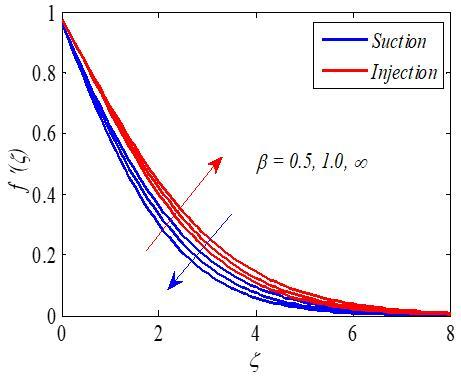Figure 2. Effect of $\beta$ on $f'\left( \zeta \right)$

## 3.jpg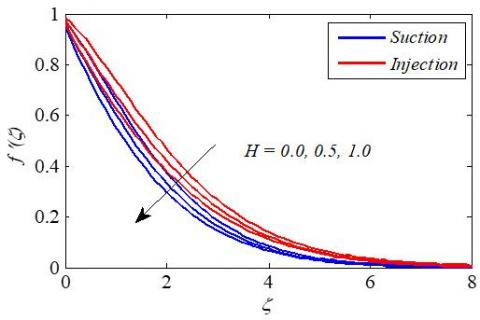Figure 3. Effect of $H$ on $f'\left( \zeta \right)$

Figure 2 is sketched to mention the repercussions of Casson parameter $\beta$ on velocity distribution $f\,'\left( \zeta \right)$. It is elucidated that velocity drops with the increase of Casson parameter for suction $\left( S=0.3 \right)$. This is because of plastic dynamic viscosity which reduces the fluid motion enhances with the Casson parameter. Opposite trend is observed for injection $\left( S=-0.3 \right)$. Larger values of magnetic parameter $H$ resemble an increase in Lorentz force which opposes the fluid motion. Hence velocity declines with magnetic parameter for both the cases. This reduction is more in suction then injection. This is shown in Figure 3. It is found that accelerating the porosity parameter $K$, devitalize the thickness of velocity field. This is because the holes of the porous layers expand with an increase in the porosity parameter and diminish the thickness of the momentum boundary layer. This is shown in Figuer 4.

## 4.jpgFigure 4. Effect of $K$ on $f'\left( \zeta \right)$

## 5.jpg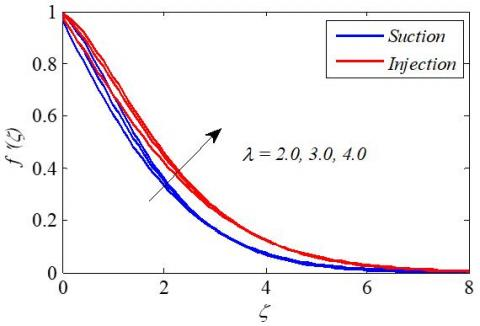Figure 5. Effect of $\lambda$ on $f'\left( \zeta \right)$

## 6.jpgFigure 6. Effect of $\delta$ on $f'\left( \zeta \right)$

Figures 5 and 6 demonstrate the impact of buoyancy parameter $\lambda$ and solutal buoyancy parameter $\delta$ on velocity. It is understood that velocity sketch upturns by enhancing the values of $\lambda$ and  $\delta$. It is also noticed that this enhancement in the velocity filed is more on injection case compared with the suction case. The Angle of inclination $\alpha$ decreases the effect of the buoyancy force due to thermal diffusion by a factor of $\cos \,\alpha$. Hence velocity decreases with $\alpha$ for both the cases. This is shown in Figure 7. Effects of velocity slip ${{S}_{v}}$ on the velocity profiles are shown in Figure 8. It is observed that the velocity increases with ${{S}_{v}}$ up to certain value of $\zeta =1.8$ for both the cases. After that velocity increases slightly with ${{S}_{v}}$. Temperature profiles are displayed for various values of radiation parameter in Figure 9. It is observed that the radiation parameter $R$ uplifts the temperature distribution. This is because of the fact that the thermal boundary layer thickness improves with increasing radiation parameter for both the cases. It is also observed that accretion in the temperature field is considerably large in injection case. The deviation of Prandtl number $\Pr$ on temperature is explained in Figure 10. It is evident from figure that enhancing the values of Prandtl numbers $\Pr$ diminishes temperature profile. For lower Prandtl numbers refers to thermal diffusion and so momentum boundary layer structures thicker, therefore the heat can drawn-out from the sheet faster than advanced values of Prandtl number $\Pr$. Figures 11 and 12 display the influence of thermophoresis parameter $Nt$ and Brownian motion parameter $Nb$ on temperature. It is clear that thermophoresis parameter $Nt$ and Brownian motion parameter $Nb$ boost up the temperature profiles. The repercussion of Eckert number $Ec$ on temperature is initiated in Figure 13. It is observed that the temperature rises with the increase of $Ec$.

## 7.jpgFigure 7. Effect of $\alpha$ on $f'\left( \zeta \right)$

## 8.jpgFigure 8. Effect of ${{S}_{v}}$ on $f'\left( \zeta \right)$

## 9.jpg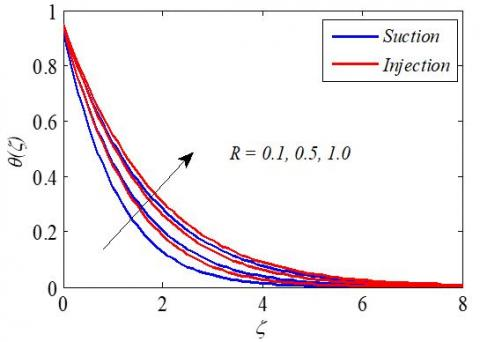Figure 9. Effect of $R$ on $\theta \left( \zeta \right)$

## 10.jpgFigure 10. Effect of $\Pr$ on $\theta \left( \zeta \right)$

## 11.jpg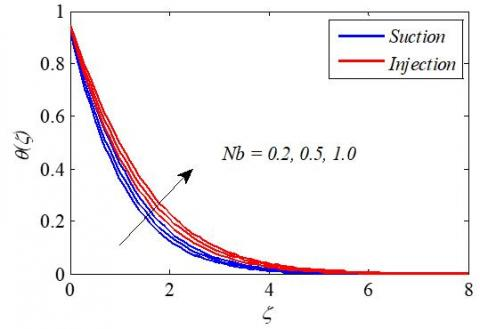Figure 11. Effect of $Nb$ on $\theta \left( \zeta \right)$

## 12.jpg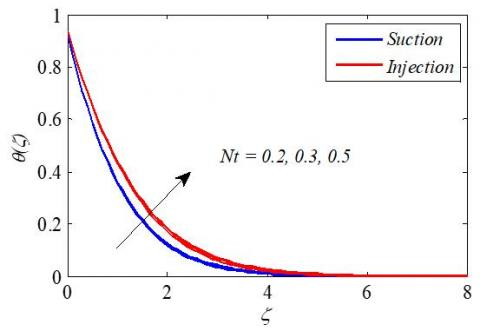Figure 12. Effect of $Nt$ on $\theta \left( \zeta \right)$

## 13.jpgFigure 13. Effect of $Ec$ on $\theta \left( \zeta \right)$

## 14.jpg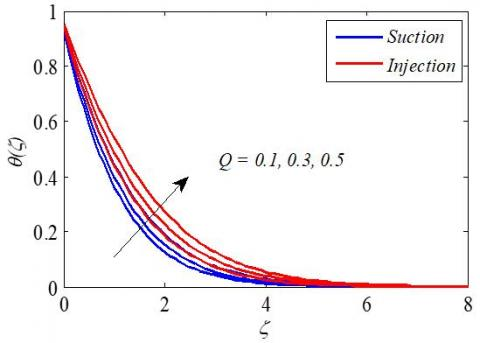Figure 14. Effect of $Q$ on $\theta \left( \zeta \right)$

## 15.jpg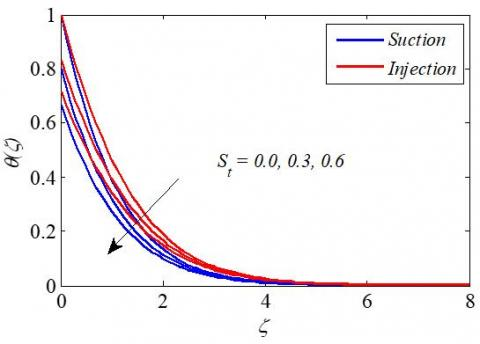Figure 15. Effect of ${{S}_{t}}$ on $\theta \left( \zeta \right)$

## 16.jpgFigure 16. Effect of $Nb$ on $\phi \left( \zeta \right)$

Figure 14 shows the effect of heat source parameter $Q$ on temperature. The figure declares that the temperature accelerates for various values of $Q$  due to heat generation exist in the thermal boundary layer and hence temperature increases. This acceleration is more for injection case. Figure 15 gives variation of temperature profiles for different values of thermal slip parameter ${{S}_{t}}$. From this figure we infer that, the temperature of the boundary layer reduces with an increase in the thermal slip parameter. Concentration profile for various values of Brownian motion parameter $Nb$ is shown in Figure 16. We noticed that the concentration profile decelerates with an increase in Nb for both cases.

## 17.jpg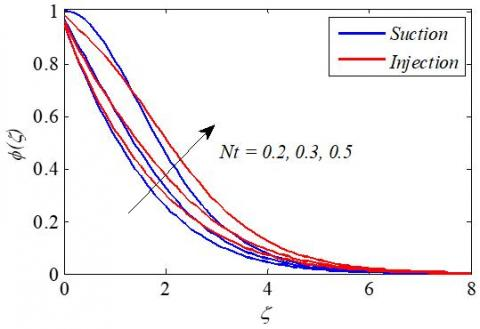Figure 17. Effect of $Nt$ on $\phi \left( \zeta \right)$

## 18.jpgFigure 18. Effect of $Sc$ on $\phi \left( \zeta \right)$

## 19.jpgFigure 19. Effect of $\gamma$ on $\phi \left( \zeta \right)$

Figure 17 has been plotted to demonstrate the effects of the thermophoresis parameter $Nt$ on the dimensionless concentration profile. We noticed that the concentration profile increase with an increasing in the value of Nt for suction and injection. Effect of Schmidt number $Sc$ on concentration distribution is displayed in Figure 18. Here concentration profile decreases when $Sc$ increases. Physically the Schmidt number is dependent on mass diffusion ${{D}_{B}}$ and an increase in $Sc$ corresponds to a decrease in mass diffusion and the concentration. Figure 19 exposes the variation of chemical reaction parameter $\gamma$ on concentration profile. It is shows that concentration profile decreases with increase in the values of $\gamma$. The effect of solutal slip parameter ${{S}_{c}}$ on the concentration profiles is plotted in Figure 20. It reveals that the concentration profiles decrease with increase in ${{S}_{c}}$.

## 20.jpg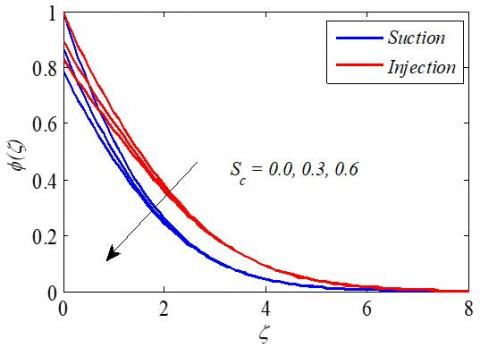Figure 20. Effect of ${{S}_{c}}$ on $\phi \left( \zeta \right)$

## 21.jpg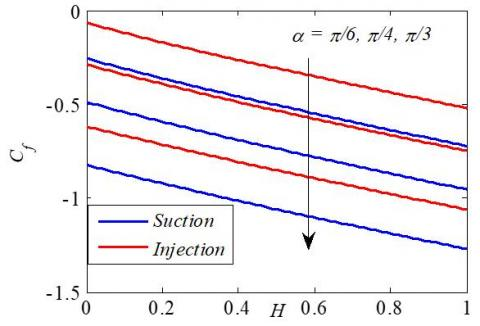Figure 21. Effect of $H$ and $\alpha$ on skin friction coefficient

## 22.jpgFigure 22. Effect of $R$ and $\lambda$ on local Nusselt number

Figure 21 is initiated to know the impact of magnetic parameter $H$ and inclination parameter $\alpha$ on skin friction coefficient. It is clear that the skin friction declines with both $H$ and $\alpha$. From Figures 22 and 23, we infer that heat transfer rate decreases with viscous dissipation $Ec$ and Brownian motion parameter $Nb$. It is also noticed that heat transfer rate increases with radiation parameter $R$and buoyancy parameter $\lambda$for both the cases. Figure 24 displays the impact of solutal slip parameter ${{S}_{c}}$ and Schmidt number $Sc$on mass transfer rate. Local Sherwood number increases with an increase in $Sc$and ${{S}_{c}}$. To endorse the veracity of the present analysis, obtained results of $-f\,'\,'\left( 0 \right)$ and $-\theta \,'\left( 0 \right)$ have been compared with the existing solutions in Tables 2 and 3.

## 23.jpgFigure 23. Effect of $Ec$ and $Nb$ on local Nusselt number

## 24.jpgFigure 24. Effect of $Sc$ and ${{S}_{c}}$ on local Sherwood number

Table 2. Comparison of $-\theta '(0)$ for different values of $M,\,\Pr ,\,Ec\,\,\text{and}\,\,R$when $\beta \rightarrow \infty, \lambda=\delta=H=K=N t=N b=S=S_{v}=S_{t}=S_{c}=0.0, Q=M=0.0$

 $Ec$ $R$ $\Pr$ Ishak  Seini and Makinde  HAM 0.0 0.0 1.0 0.9547 0.954811 0.954783 0.0 0.0 3.0 1.8691 1.869069 1.869067 0.0 1.0 1.0 0.5315 -- 0.531503 0.9 0.0 1.0 0.5385 -- 0.538541 0.9 0.0 3.0 0.8301 -- 0.830137 0.9 1.0 1.0 0.3343 -- 0.334521 0.9 1.0 3.0 0.6055 -- 0.605519

Table 3. Comparison of $-\theta '\left( 0 \right)$ and $\phi '\left( 0 \right)$ for different values of $\beta$ when$\lambda=\delta=3.0, H=K=1.0, N t=N b=0.5, S=0.7, R=1.0$, $\operatorname{Pr}=0.72, S_{v}=0.1, S_{t}=0.3, \quad S_{c}=0.7, \gamma=1.0, \alpha=\pi / 4, E c=Q=0.0$

 Eswara Rao  HAM $\beta$ $-\theta \,'\left( 0 \right)$ $-\phi \,'\left( 0 \right)$ $-\theta \,'\left( 0 \right)$ $-\phi \,'\left( 0 \right)$ 0.3 0.523109 0.589403 0.523113 0.589411 0.6 0.512534 0.588060 0.512534 0.588060
6. Conclusions

In this paper, we obtained the numerical analysis of magneto-hydrodynamic Casson nanofluid flow at an exponentially inclined stretching plate, considering thermal radiation, heat source, viscous dissipation and chemical reaction. The main findings of computational results are summarized below:

• Velocity is an increasing function of the parameters $\lambda$ and $\delta$.
• Temperature profile falls down against Prandtl number and thermal slip parameter, while Brownian motion parameter, thermophoresis number and radiation parameter enhances it.
• Concentration profile reduces against Brownian motion parameter and Schmidt number while it enhances for thermophoresis number.
• Local Nusselt number enhances for buoyancy parameter.
• By increasing solutal slip parameter local Sherwood number enhances.
Nomenclature
 $B$ variable magnetic field strength, N. m-1.A-1 ${{B}_{0}}$ constant $\text{C}$ nanoparticle concentration, kg. m-3 ${{C}_{0}}$ reference concentration ${{C}_{f}}$ skin friction coefficientlocal ${{C}_{w}}$ nanoparticle concentration at the surface of the sheet ${{C}_{\infty }}$ ambient nanoparticle concentration ${{D}_{B}}$ Brownian diffusion coefficient, m2. s-1 ${{D}_{T}}$ thermophoretic diffusion coefficient,   m2. s-1 $Ec$ viscous dissipation parameter, W. m-2 $Gr$ local grashof number $Gc$ local solutal grashof number $H$ Hartman number ${{J}_{w}}$ mass flux ${{K}^{*}}$ permeable parameter $K$ non-dimensional porous parameter $L$ reference length ${{M}^{*}}$ constant $M$ thermal slip parameter ${{N}^{*}}$ constant $N$ velocity slip parameters $Nb$ Brownian motion parameter $Nt$ thermophoresis parameter $N{{u}_{x}}$ local Nusselt number ${{P}^{*}}$ constant $P$ solutal slip parameter ${{P}_{y}}$ yield stress, Nm-2 $\Pr$ Prandtl number ${{Q}_{0}}$ heat source coefficient $Q$ heat source parameter $R$ radiation parameter ${{\operatorname{Re}}_{x}}$ local Reynolds number ${{q}_{r}}$ radiative heat flux, W. m-1 ${{q}_{w}}$ surface  heat flux $S$ suction/injection parameter ${{S}_{v}}$ non dimensional velocity slip parameter ${{S}_{t}}$ non dimensional thermal slip parameter ${{S}_{c}}$ non dimensional solutal slip parameter $Sc$ Schmidt number $S{{h}_{x}}$ Sherwood number $T$ Temperature, K ${{T}_{0}}$ reference temperature, K ${{T}_{w}}$ surface temperature, K ${{T}_{\infty }}$ ambient fluid temperature, K $U$ stretching velocity, m. s-1 ${{U}_{0}}$ reference velocity, m. s-1 $u,\,v$ velocity components in $x,\,y,$ directions, m. s-1 $V$ velocity at the wall, m.s-1 Greek symbols $\text{ }\!\!\nu\!\!\text{ }$ kinematic viscosity, m2. s-1 $\text{ }\!\!\sigma\!\!\text{ }$ electrical conductivity of the fluid, S. m-1 $\rho$ fluid density, kg. m-3 $\alpha$ inclination angle ${{\tau }_{w}}$ surface shear rate, Pa $\beta$ Casson fluid parameter ${{h}_{f}}$ convective heat transfer coefficient of the fluid $k$ thermal conductivity of the fluid, W. m-1. k-1 ${{\sigma }^{*}}$ Stefan-Boltzman constant, W. m-2. K-4 ${{c}_{p}}$ specific at constant pressure, J.K-1.kg-1 $p$ fluid pressure, Pa ${{\mu }_{B}}$ plastic dynamic viscosity of the fluid, Pa. s $g$ acceleration due to gravity, m. s-2 $f\,'$ velocity profiles, m. s-1 $\theta$ temperature profile $\phi$ concentration profile ${{k}^{*}}$ mean absorption coefficient, m-1 $\zeta$ similarity variable $\Gamma$ chemical reaction rate $\lambda$ buoyancy parameter $\delta$ solutal buoyancy parameter $\gamma$ chemical reaction parameter ${{\beta }_{T}}$ coefficient of thermal expansion ${{\beta }_{C}}$ coefficient of solutal expansion Subscript   s $w$ conditions at the wall $\infty$ ambient condition
References

 Gupta, P.S., Gupta, A.S. (1977). Heat and mass transfer on a stretching sheet with suction or blowing. Canadian Journal of Chemical Engineering, 55(6): 744-746. https://doi.org/10.1002/cjce.5450550619

 Cortell, R. (2007). Viscous flow and heat transfer over a nonlinearly stretching sheet. Applied Mathematics and Computation, 184(2): 864-873. https://doi.org/10.1016/j.amc.2006.06.077

 Shahzad, A., Ali, R., Khan, M. (2012). On the exact solution for axisymmetric flow and heat transfer over a non radially stretching sheet. Chinese Physics Letter, 29(8): 084705. https://doi.org/10.1088/0256-307X/29/8/084705

 Tiwari, R.K., Das, M.K. (2007). Heat transfer augmentation in two sided lid driven differentially heated square cavity utilizing nanofluids. International Journal of Heat and Mass Transfer, 50(9-10): 2002-2018. http://doi.org/10.1016/j.ijheatmasstransfer.2006.09.034

 Abba, Z., Hayat, T. (2011). Stagnation slip flow and heat transfer over a nonlinear stretching sheet. Numerical Methods for Partial Differential Equations, 27(2): 302-314. https://doi.org/10.1002/num.20523

 Hayat, T., Qasim, M., Mesloub, S. (2011). MHD flow and heat transfer over permeable stretching sheet with slip conditions. Numerical Methods in Fluids, 66(8): 963-975. https://doi.org/10.1002/fld.2294

 Cortell, R. (2014). Fluid flow and radiative nonlinear heat transfer over a stretching sheet. Journal of King Saud University-Science, 26(2): 161-167. https://doi.org/10.1016/j.jksus.2013.08.004

 Choi, S.U.S., Eastman, J.A. (1995). Enhancing thermal conductivity of fluids with nanoparticles. ASME International Mechanical Engineering Congress Exposition SanFrancisco, USA, ASME, FED231/MD66, pp. 99-105.

 Makinde, O.D., Aziz, A. (2011). Boundary layer flow of a nanofluid past a stretching sheet with a convective boundary condition. International Journal of Thermal Science, 50(7): 1326-1332. https://doi.org/10.1016/j.ijthermalsci.2011.02.019

 Mansur, S., Ishak, A. (2013). The flow and heat transfer of a nanofluid past a stretching/shrinking sheet with a convective boundary condition. Abstract and Applied Analysis, 2013(2): 350647. https://doi.org/10.1155/2013/350647

 Mabood, F., Khan, W.A., Ismail, A.I.M. (2015). MHD boundary layer flow and heat transfer of nanofluids over a nonlinear stretching sheet: A numerical study. Journal of Magnetism and Magnetic Materials, 374: 569-576. https://doi.org/10.1016/j.immm.2014.09.013

 Hady, F.M., Ibrahim, F.S., Abdel-Gaied, S.M., Eid, M.R. (2012). Radiation effect on viscous flow of a nanofluid and heat transfer over a nonlinearly stretching sheet. Nanoscale Research Letter, 7: 229. https://doi.org/10.1186/1556-276X-7-229

 Nadeem, S., Ul Haq, R., Khan, Z.H. (2014). Numerical solution of non-Newtonian nanofluid over a stretching sheet. Applied Nanoscience, 4(5): 625-631. https://doi.org/10.1007/s13204-013-0235-8

 Mustafa, M., Hayat, T., Pop, I., Asghar, S., Obaidat, S. (2011). Stagnation-point flow of a nanofluid towards a stretching sheet. International Journal of Heat and Mass Transfer, 54(25-26): 5588-5594. https://doi.org/10.1016/j.ijheatmasstransfer.2011.07.021

 Hamad, M.A.A., Ferdows, M. (2012). Similarity solutions to viscous flow and heat transfer of nanofluid over nonlinearly stretching sheet. Applied Mathematics and Mechanics, 33(7): 923-930. https://doi.org/10.1007/s10483-012-1595-7

 Jayarami Reddy, K., Madhusudhan, N.P., Ramakrishna, K. (2018). MHD mixed convection flow of radiating and chemically reactive Casson nanofluid over a nonlinear permeable stretching sheet with viscous dissipation and heat source. Multidiscipline Modelling in Materials and Structures, 14(3): 609-630. https://doi.org/10.1108/MMMS-10-2017-0127

 Jayarami Reddy, K., Madhusudhan, N.P., Ramakrishna, K., Abhishek. D. (2018). Numerical investigation of chemical reaction and heat source on radiating MHD stagnation point flow of carreau nanofluid with suction/injection. Defect and Diffusion Forum, 388: 171-189. https://doi.org/10.4028/www.scientific.net.DDF.388.171

 Suneetha, K., Ibrahim, S.M., Jayarami Reddy, K. (2015). Effects of radiation, viscous and joules dissipation on MHD mixed convective flow in porous medium in Presence of heat source, chemical reaction and Soret. International Journal of Scientific and Innovative Mathematical Research (IJSIMR), 3(2): 769-779.

 Mustafa, M., Khan, J.A. (2015). Model for flow of Casson nanofluid past a non-linearly stretching sheet considering magnetic effects.AIP Advances, 5: 077148. https://doi.org/10.1063/1.4927449

 Ibrahim, W., Makinde, O.D. (2016). Magnetohydrodynamic stagnation point flow and heat transfer of Casson nanofluid past a stretching sheet with slip and convective boundary conditions. Journal of Aerospace Engineering, 29(2): 04015037. https://doi.org/10.1061/(ASCE)AS.1943-5525.0000529

 Ibrahim, S.M., Kumar, P.V., Lorenzini, G., Mabood. F.(2017). Numerical study of the onset of chemical reaction and heat source on dissipative MHD stagnation point flow of Casson nanofluid over a nonlinear stretching sheet with velocity slip and convective boundary conditions. Journal of Engineering Therophysics, 26(2): 256-271. https://doi.org/11.1134/S18102328170200096

 Ibrahim, S.M., Lorenzini, G., Vijaya Kumar, P., Raju, C.S.K. (2017). Influence of chemical reaction and heat source on dissipative MHD mixed convection flow of a Casson nanofluid over a nonlinear permeable stretching sheet. International Journal of Heat and Mass Transfer, 111: 346-355. https://doi.org/10.1016/j.ijheatmasstransfer.2017.03.097

 Mondal, M., Biswas, R., Shanchia, K., Hassan, M., Ahmad, S.F. (2018). Numerical investigation with stability convergence analysis of chemically hydromagnetic Casson nanofluid flow in the effects of thermophoresis and brownian motion. International Journal of Heat and Technology, 37(1): 59-70. https://doi.org/10.18280/ijht.370107

 Kumar, P.V., Ibrahim, S.M., Lorenzini, G. (2018). The study of three dimensional radiative MHD Casson nanofluid over an exponentially porous stretching sheet with heat source under convective boundary conditions. International Journal of Heat and Technology, 36(1): 1-10. https://doi.org/10.18280/ijht.360101

 Kumar, P.V., Ibrahim, S.M., Lorenzini, G. (2018). Impact of thermal radiation and Joule heating on MHD mixed convection flow of a Jeffrey fluid over a stretching sheet using homotopy analysis method. International Journal of Heat and Technology, 35(4): 978-986. https://doi.org/10.18280/ijht.350434

 Hayat, T., Shehzad, S.A., Alsaedi, A. (2012). Soret and Dufour effects on Magnetohydrodynamic (MHD) flow of Casson fluid. Applied Mathematics and Mechanics, 33(10): 1301-1312. https://doi.org/10.1007/s10483-012-1623-6

 Liao, S.J. (2012). Homotopy Analysis Method in Nonlinear Differential Equations. Springer & Higher Education Press, Heidelberg.

 Nadeem, S., Zaheer, S., Fang, T. (2011). Effects of thermal radiation on the boundary layer flow of a Jeffrey fluid over an exponentially stretching surface. Numerical Algorithms, 57(2): 187-205. https://doi.org/10.1007/s11075-010-9423-8

 Ishak, A. (2011). MHD boundary layer flow due to an exponentially stretching sheet with radiation effect. Sains Malaysian, 40(4): 391-395.

 Seini, Y.I., Makinde, O.D. (2013). MHD boundary layer flow due to exponential stretching surface with radiation and chemical reaction. Mathematical Problems in Engineering, 2013: 163614. https://dx.doi.org/10/1155/2013/163614

 Eswara Rao, E. (2018).The effects of thermal radiation and chemical reaction on MHD flow of a Casson fluid over an exponentially inclined stretching surface. Journal of Physics: Conference Series, 012158. https://doi.org/10.1088/1742-6596/1000/1/012158